# Acyclic orientation

(diff) ← Older revision | Latest revision (diff) | Newer revision → (diff)

An orientation (assignment of direction) of each edge of a graph such that no cycle in the graph is a cycle (consistently oriented) in the resulting directed graph (cf. Graph, oriented).

An acyclic orientation of a graphcan be obtained from a proper colouringby orienting each edgefromto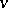if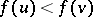(cf. Graph colouring). Given an acyclic orientationof a connected graphthat is not a forest (cf. also Graph, connectivity of a), let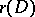be the maximum over all cycles inof, where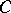has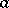forward edges and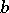backward edges in. G.J. Minty [a7] proved thatand that this is best possible (equality holds for the acyclic orientation obtained above from an optimal colouring). Minty's theorem implies the related Gallai–Roy theorem ([a5], [a9], [a14]) that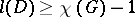whereis the maximum length of a path in an orientationof(equality holds for the orientation defined above).

R.P. Stanley [a11] proved that the number of acyclic orientations of a graphis the value at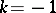of the chromatic polynomial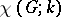(computing this number is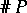-complete [a13]). More generally, suppose thatis an acyclic orientation of an-vertex graphand that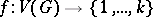is a-colouring of. One says that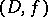is a compatible pair if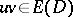implies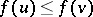. Stanley proved that the number of compatible pairs is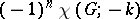. This relationship is an instance of combinatorial reciprocity. Stanley [a12] further generalized this to count acyclic orientations withsinks. C. Greene [a6] proved that the acyclic orientations of a graphare in one-to-one correspondence with the regions of an associated arrangement of hyperplanes; T. Zaslavsky [a15] has generalized this to acyclic orientations of signed graphs.

An edge in an acyclic orientation is dependent when reversing it would create a cycle. The acyclic orientation graph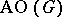is the graph whose vertices are the acyclic orientations of, with two vertices adjacent when they differ by the reversal of an edge; the degree of a vertex is its number of independent edges. Every acyclic orientation of a connected-vertex graphhas at leastindependent edges (deletion of dependent edges does not disconnect), and this is best possible (equality holds when the independent edges arise from a depth-first search tree). Thus, the minimum degree ofis. P.H. Edelman (see also [a10]) proved that the connectivity ofis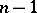.

Whenhasedges,is isomorphic to an induced subgraph of the-dimensional hypercube and is thus bipartite (cf. Graph, bipartite). It is conjectured thatis Hamiltonian when its two partite sets have the same size. G. Pruesse and F. Ruskey [a8] proved that the Cartesian product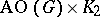is always Hamiltonian. These Hamiltonicity questions relate to the combinatorial Gray code problem (cf. Gray code) of listing the acyclic orientations by reversing one edge at a time.

When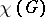is less than the girth of, the list of vertex degrees inincludes all values fromup to the maximum degree [a4]. It is unknown whether this is true for all graphs. When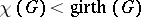, there exist acyclic orientations in which every edge is independent.

A graph has an acyclic orientation in which every edge is independent if and only if it is a cover graph; this acyclic orientation is the cover relation of a partially ordered set (cf. Partial order). Recognition of cover graphs is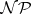-complete. The smallest triangle-free graph with chromatic number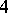(violating the condition) is the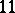-vertex Grötzsch graph, and it is not a cover graph.

Other types of acyclic orientations characterize important families of graphs. An acyclic orientationof a simple graph is a transitive orientation ifand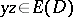together imply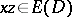. The graphs having transitive orientations are the comparability graphs of partially ordered sets. A graph is perfectly orderable if it has a vertex ordering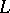such that greedily colouring the vertices of any induced subgraph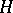in the order specified byuses only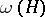colours, whereis the size of the largest clique in. V. Chvátal [a3] proved thatis perfectly orderable if and only if it has an acyclic orientation having no induced-vertex path with both end-edges oriented outward.

Letbe the worst-case number of edges that must be probed by an algorithm that finds an unknown acyclic orientation of. A graph is exhaustive if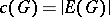. Graphs having an acyclic orientation in which every edge is independent are exhaustive. For bounds on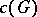in terms of the independence number ofsee [a1]. N. Alon and Zs. Tuza [a2] proved that for the random graph with constant edge probability, almost surely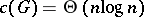. Both papers also study exhaustive graphs.

Acyclic orientations have computational applications in percolation, network reliability, neural networks, parallel sorting, scheduling, etc.

How to Cite This Entry:
Acyclic orientation. Encyclopedia of Mathematics. URL: http://encyclopediaofmath.org/index.php?title=Acyclic_orientation&oldid=11691
This article was adapted from an original article by D. West (originator), which appeared in Encyclopedia of Mathematics - ISBN 1402006098. See original article